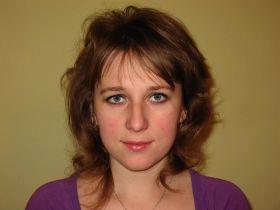##Education: Ivan Franko National University of Lviv (specialtymathematics, 2006.)

Scientific degree: Ph.D. Degree (01.01.06 algebra and number theory, 2019) ( thesis: "Equivalence of matrices over quadratic rings and matrix equations")

Position: Junior Research Fellow (2019)

In Institute: from 2006

Research interests: algebra and number theory

Major publications:

1. The number of standard forms of matrices over imaginary Euclidean quadratic rings with respect to the (z,k)-equivalence (with Petrychkovych V.M.) // Mat. Stud. – 2022. – 57, No. 2. – 7 p.
2. Standard form of matrices over quadratic rings with respect to the (z,k)-equivalence and the structure of solutions of bilateral matrix linear equations (with Petrychkovych V.M.) // J. Math. Sci. 2021.  253, (1). P. 5462.
3. The standard form of matrices over the ring of gaussian integers with respect to (z,k)-equivalence (with Petrychkovych V.M. and Zelisko H. V.) // Appl. probl. mech. and math. 2020 –№18. P.5 10.
4. Matrix Diophantine equations over quadratic rings and their solutions (with Petrychkovych V.M. and Zelisko H. V.) // Carpathian Math. Publ. − 2020. −12, №2. – Р. 368–375.
5. Matrix linear unilateral and bilateral equations over quadratic rings (with Petrychkovych V.M.) // Visnyk of the Lviv Univ. Series Mech. Math. 2018.  85. P. 3240. (in Ukrainian).
6. The integer solutions of matrix linear unilateral and bilateral equations over quadratic rings // J. Math. Sci. 2017.  223, (1). P. 5059.
7. Equivalence of pairs of matrices with relatively prime determinants over quadratic rings of principal ideals (with Petrychkovych V.M.) //  Bul. Acad. de  Ştiinţe Repub.  Mold.  Mat. 2014.  3, (76) P. 38–48.
8. On equivalence of pairs of matrices, which determinants are primes powers, over quadratic Euclidean rings // Carpathian Math. Publications. – 2013. ­  5, (1). P. 63–69. (in Ukrainian).
9. On equivalence of matrices over quadratic Euclidean rings (with Petrychkovych V.M., Zelisko V.R.) // Appl. probl. mech. and math.. 2006. № 4. P. 16–21. (in Ukrainian).

List of Conference Proceedings:

1. Natalija Ladzoryshyn, Vasyl' Petrychkovych. On the number of standard forms of matrices over imaginary Euclidean quadratic rings with respect to the (z,k)-equivalence // The 13th International Algebraic Conference in Ukraine, July 69, 2021, Taras Shevchenko National University of Kyiv. – Book of Abstracts. – P. 45.
2. Ladzoryshyn N., Petrychkovych V. The standard form of matrices over imaginary Euclidean quadratic rings with respect to (z,k)-equivalence // XI International Skorobohatko Mathematical Conference. October 26−30, 2020, Lviv, Ukraine. Abstracts: Lviv: Pidstryhach Institute for Applied Problems of Mechanics and Mathematics, 2020. – 130 p. – – [Electronic resource]. – Access mode: http://www.iapmm.lviv.ua/conf_skorob2020/documents/2020tezy.pdf – P. 59.
3. Ladzoryshyn N. (z,k)-equivalence of matrices over the ring of Gaussian integers // Conference of young Scientists “Pidstryhach reading – 2020”. – L’viv, Ukraine May 26–28, 2020, L’viv. – [Electronic resource]. – Access mode: (http://www.iapmm.lviv.ua/chyt2020/abstracts/Ladzoryshyn.pdf).
4. Ladzoryshyn N., Petrychkovych V. (z,k)-equivalence of matrices over Euclidean quadratic rings and solutions of matrix equation AX+YB=C // Book of abstracts of the XII International Algebraic Conference in Ukraine dedicated to the 215th anniversary of  V. Bunyakovsky, July 0206, 2019, Vinnytsia, 2019. – P. 63–64.
5. Ladzoryshyn N. Solutions of the matrix equation AX+BY=C over Euclidean quadratic rings // Conference of young Scientists Pidstryhach reading – 2019. Lviv, Ukraine, May 2729, 2019, Lviv. [Electronic resource]. – Access mode: ().
6. Ladzoryshyn N. The solvability of Diophantine equations over quadratic rings // Modern problems of Mechanics and Mathematics: collection of scientific papers in 3 vol. / Edited by A.М. Samoilenko, R.M. Kushnir [Electronic resource] // Pidstryhach Institute for Applied Problems of Mechanics and Mathematics NAS of Ukraine. – 2018. – Vol. 3. – Access mode: (http://www.iapmm.lviv.ua/mpmm2018/Volume_3.pdf). P. 217–218.
7. Ladzoryshyn N.B. The matrix linear unilateral equations over quadratic rings // Conference of young Scientists Pidstryhach reading2015. L’viv, Ukraine, May 2628, 2015, L’viv. [Electronic resource]. – Access mode (http://www.iapmm.lviv.ua/chyt2015/theses/Ladzoryshyn.pdf).
8. Ladzoryshyn N., Petrychkovych V. On solutions of the matrix linear equations AX+YB=C over quadratic rings // Book of abstracts of the X International Algebraic Conference in Ukraine dedicated to the 70th anniversary of Yu. A. Drozd, August 20 – 27, 2015, Odessa. – Abstract. – Odessa: TES, 2015. – P. 61.
9. Ladzoryshyn N.B. Integer solutions of matrix Diophantine equation over quadratic rings // Conference of young Scientists Pidstryhach reading2014. L’viv, Ukraine, May 2830, 2014, L’viv. [Electronic resource]. – Access mode (http://www.iapmm.lviv.ua/chyt2014/theses/Ladzoryshyn.pdf).
10.  Ladzoryshyn N.B. The integral solutions of bilateral linear matrix equations over quadratic rings //  International Algebraic Conference dedicated to 100th anniversary of L.A. Kaluzhnin. Book of Abstracts.    Jule 7–12, 2014. Kyiv.   P. 52.
11. Ladzoryshyn N. The matrix linear bilateral equations over quadratic rings  // International ConferenceModern Problems of Mechanics and Mathematics”. Lviv, Ukraine May 21–25, 2013, Lviv. Abstract. – L’viv: YA. S. Pidstryhach Institute for APMM NAS of Ukraine. – P. 187–189.
12. Ladzoryshyn N., Petrychkovych V. Equivalence of pairs of matrices with relatively prime determinants over quadratic principal ideal rings // 9-th International algebraic conference in Ukraine, L'viv, July 813, 2013: Book of abstracts. – L'viv, 2013. – P. 109.
13. Ladzoryshyn N.B. On equivalence of certain pairs of matrices over quadratic Euclidean rings // XIII inter­natio­nal Scientific Kravchuk mathematical conference. – Kyiv, Ukraine , May 13–15, 2010: Conference materials. V.2 – K. NTUU – 320 p. – P. 166.
14. Ladzoryshyn N.B. On equivalence of pairs of matrices over quadratic Euclidean rings // Conference of young Scientists Pidstryhach with Modern Problems of Mechanics and Mathematics. – Lviv, Ukraine, May 25–27, 2009. – P. 176–177.
15. Volodymyr Zelisko, Natalija Ladzoryshyn, On equivalence and factorization of matrices over quadratic rings // 6th International Algebraic Conference in Ukraine.Lviv, Ukraine, July 1–7, 2007. – P.231–232.

Phone service: (032) 258 96 22